2019-09-29 09:44:19 weixin_43392489 阅读数 135
• ###### 携手STM32CubeMX玩转STM32

795 人正在学习 去看看 李凯龙

图像的傅里叶频谱特性分析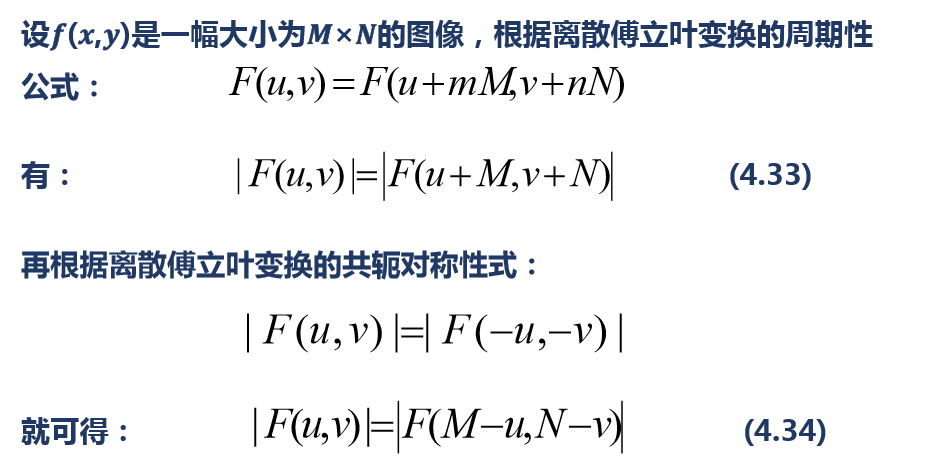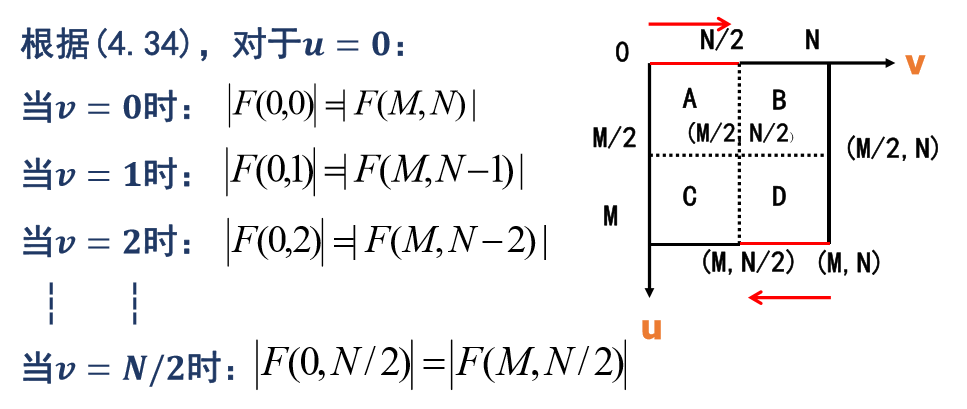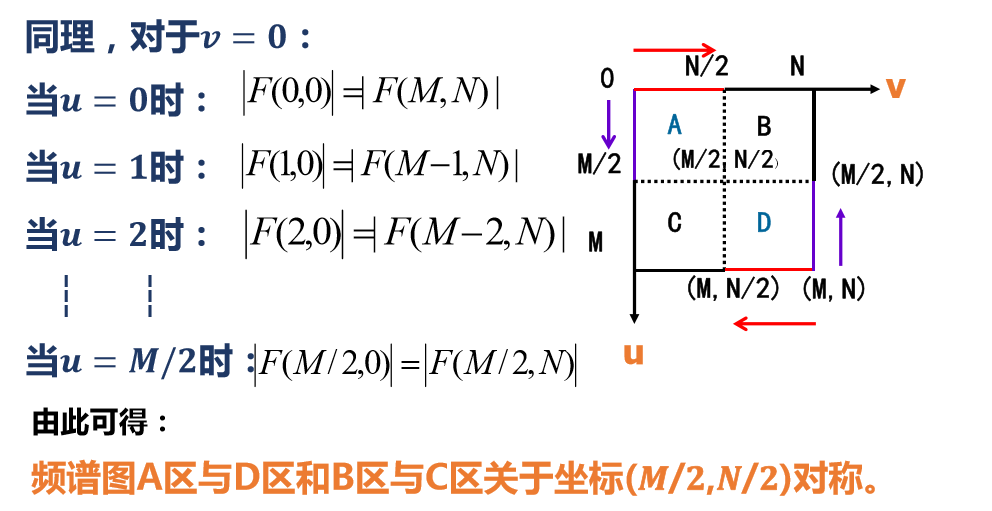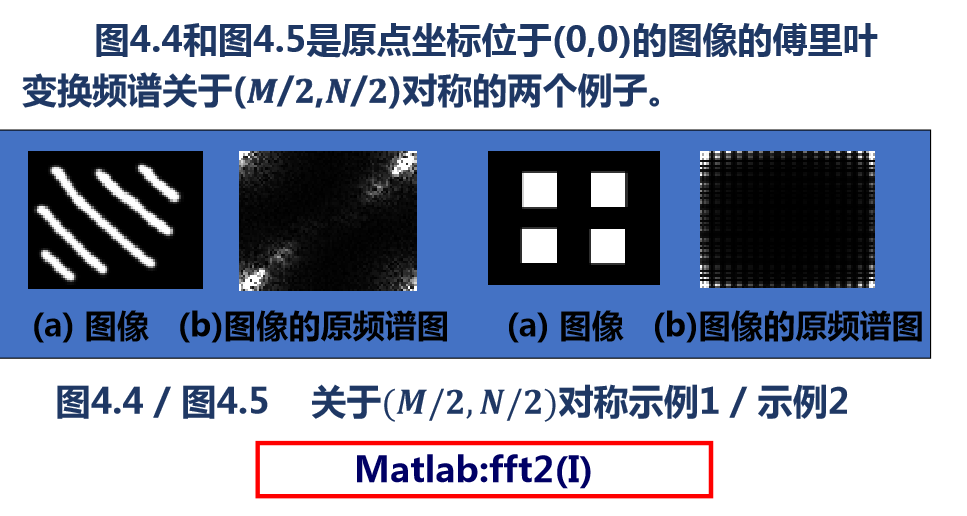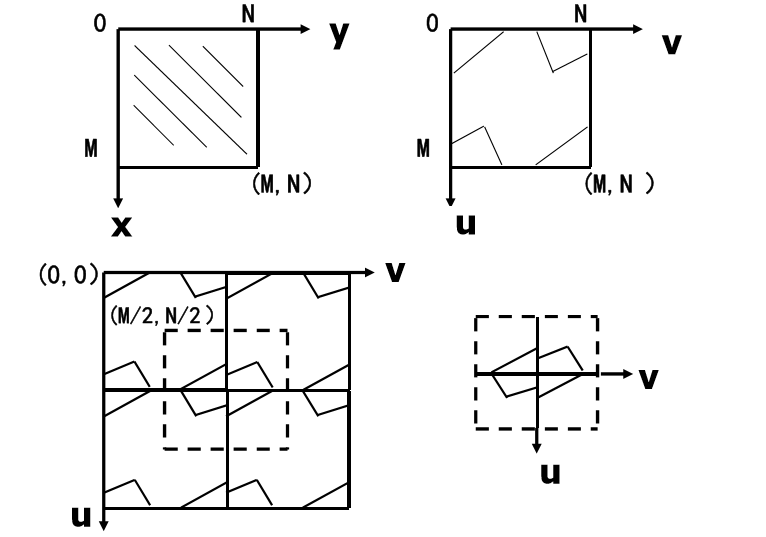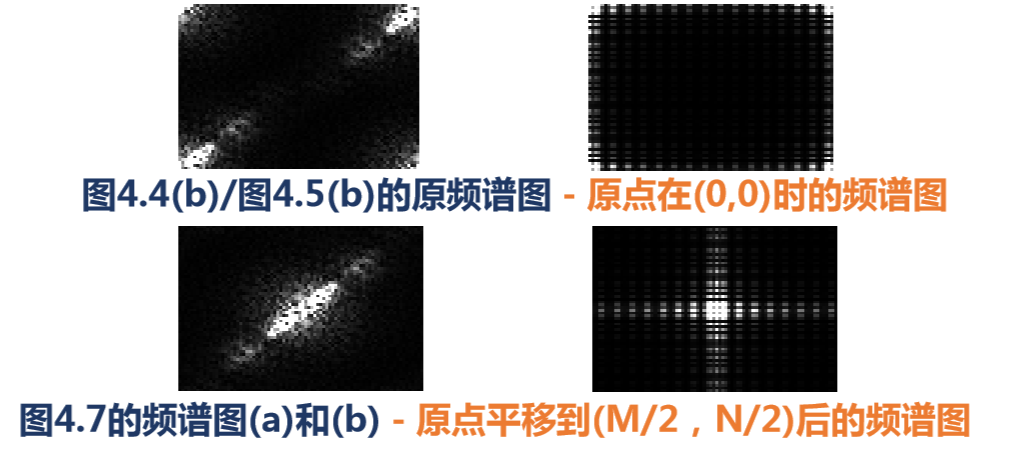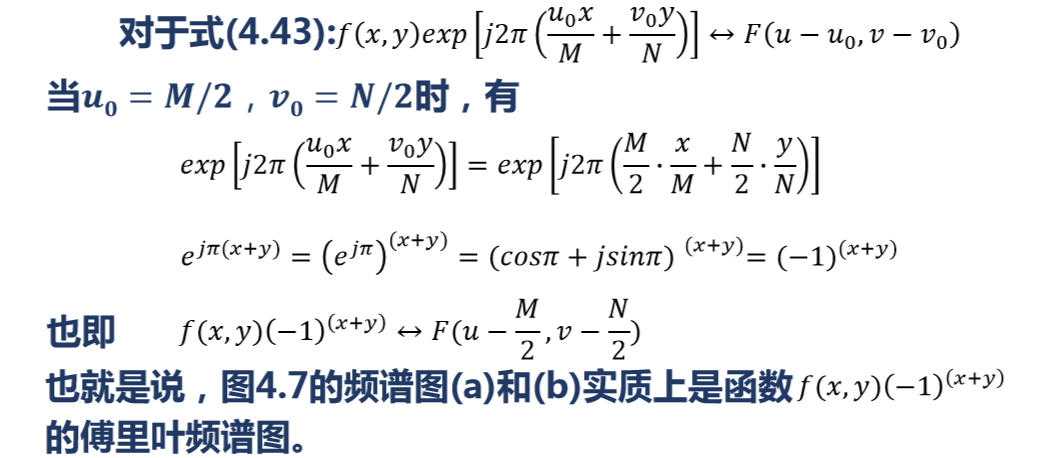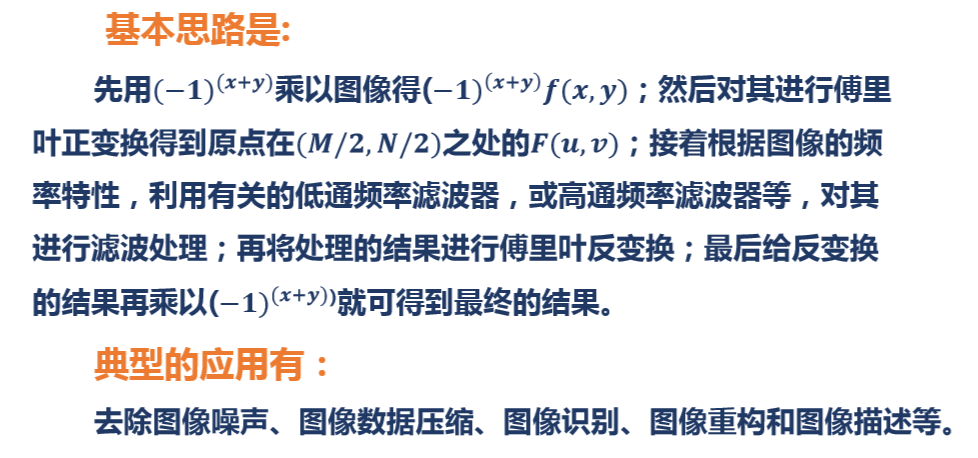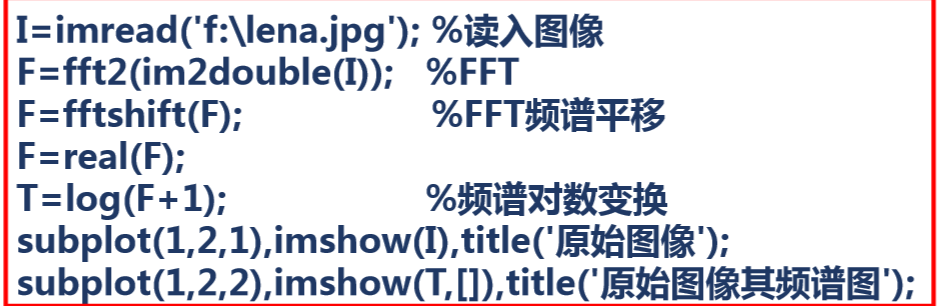2017-03-25 16:47:59 yimingsilence 阅读数 1942
• ###### 携手STM32CubeMX玩转STM32

795 人正在学习 去看看 李凯龙

# 2. 傅里叶频谱的计算

void cvDFT( const CvArr* src, CvArr* dst, int flags, int nonzero_rows=0 )

|F(u,v)|=R2(u,v)+F2(u,v)2

IplImage* fft2(IplImage* image_input)
{
int dftWidth  = getOptimalDFTSize(image_input->width);
int dftHeight = getOptimalDFTSize(image_input->height);

//cout<< " Width" <<  image_input->width << "    " <<  dftWidth  << "\n";
//cout<< "Height" << image_input->height << "    " <<  dftHeight << "\n";

IPL_DEPTH_8U,
1);

IplImage *image_Re =0 , *image_Im = 0, *image_Fourier = 0;

image_Re = cvCreateImage(cvSize(dftWidth,dftHeight),IPL_DEPTH_64F,1);
image_Im = cvCreateImage(cvSize(dftWidth,dftHeight),IPL_DEPTH_64F,1);
image_Fourier = cvCreateImage(cvSize(dftWidth,dftHeight),IPL_DEPTH_64F,2);

//image_Im <--- 0
cvZero(image_Im);
//image_Fourier <--- image_Re
//image_Fourier <--- image_Im
cvMerge(image_Re,image_Im,0,0,image_Fourier);

cvDFT(image_Fourier,image_Fourier,CV_DXT_FORWARD);

//image_Fourier ---> image_Re
//image_Fourier ---> image_Im
cvSplit(image_Fourier,image_Re,image_Im,0,0);

//Mag = sqrt(Re^2 + Im^2)
cvPow(image_Re,image_Re,2.0);
cvPow(image_Im,image_Im,2.0);
cvPow(image_Re,image_Re,0.5);

// log (1 + Mag)
cvLog (image_Re,image_Re);

//  |-----|-----|           |-----|-----|
//  |  1  |  3  |           |  4  |  2  |
//  |-----|-----|   --->    |-----|-----|
//  |  2  |  4  |           |  3  |  1  |
//  |-----|-----|           |-----|-----|

IplImage *Fourier = cvCreateImage(cvSize(dftWidth,dftHeight),IPL_DEPTH_64F,1);
cvZero(image_Fourier);

int cx = image_Re->width/2;
int cy = image_Re->height/2;

cvSetImageROI(image_Re,cvRect( 0, 0,cx,cy));  // 1
cvSetImageROI( Fourier,cvRect(cx,cy,cx,cy));  // 4

cvSetImageROI(image_Re,cvRect(cx,cy,cx,cy));  // 4
cvSetImageROI( Fourier,cvRect( 0, 0,cx,cy));  // 1

cvSetImageROI(image_Re,cvRect(cx, 0,cx,cy));  // 3
cvSetImageROI( Fourier,cvRect( 0,cy,cx,cy));  // 2

cvSetImageROI(image_Re,cvRect( 0,cy,cx,cy));  // 2
cvSetImageROI( Fourier,cvRect(cx, 0,cx,cy));  // 3

cvResetImageROI(image_Re);
cvResetImageROI( Fourier);

cvNormalize(Fourier,Fourier,1,0,CV_C,NULL);

return(Fourier);
}123456789101112131415161718192021222324252627282930313233343536373839404142434445464748495051525354555657585960616263646566676869707172737475767778123456789101112131415161718192021222324252627282930313233343536373839404142434445464748495051525354555657585960616263646566676869707172737475767778

int dftWidth  = getOptimalDFTSize(image_input->width);
int dftHeight = getOptimalDFTSize(image_input->height);
IPL_DEPTH_8U,
1);
cvCopyMakeBorder( image_input, image_padded, cvPoint(0,0), IPL_BORDER_CONSTANT,cvScalarAll(0)); 123456123456

IplImage *image_Re =0 , *image_Im = 0, *image_Fourier = 0;

image_Re = cvCreateImage(cvSize(dftWidth,dftHeight),IPL_DEPTH_64F,1);
image_Im = cvCreateImage(cvSize(dftWidth,dftHeight),IPL_DEPTH_64F,1);
image_Fourier = cvCreateImage(cvSize(dftWidth,dftHeight),IPL_DEPTH_64F,2);

//image_Im <--- 0
cvZero(image_Im);
//image_Fourier <--- image_Re
//image_Fourier <--- image_Im
cvMerge(image_Re,image_Im,0,0,image_Fourier);

cvDFT(image_Fourier,image_Fourier,CV_DXT_FORWARD);

//image_Fourier ---> image_Re
//image_Fourier ---> image_Im
cvSplit(image_Fourier,image_Re,image_Im,0,0);1234567891011121314151617181912345678910111213141516171819

//Mag = sqrt(Re^2 + Im^2)
cvPow(image_Re,image_Re,2.0);
cvPow(image_Im,image_Im,2.0);
cvPow(image_Re,image_Re,0.5);

// log (1 + Mag)
cvLog (image_Re,image_Re); 123456789123456789

|F(u,v)|=R2(u,v)+F2(u,v)2

//  |-----|-----|           |-----|-----|
//  |  1  |  3  |           |  4  |  2  |
//  |-----|-----|   --->    |-----|-----|
//  |  2  |  4  |           |  3  |  1  |
//  |-----|-----|           |-----|-----|

IplImage *Fourier = cvCreateImage(cvSize(dftWidth,dftHeight),IPL_DEPTH_64F,1);
cvZero(image_Fourier);

int cx = image_Re->width/2;
int cy = image_Re->height/2;

cvSetImageROI(image_Re,cvRect( 0, 0,cx,cy));  // 1
cvSetImageROI( Fourier,cvRect(cx,cy,cx,cy));  // 4

cvSetImageROI(image_Re,cvRect(cx,cy,cx,cy));  // 4
cvSetImageROI( Fourier,cvRect( 0, 0,cx,cy));  // 1

cvSetImageROI(image_Re,cvRect(cx, 0,cx,cy));  // 3
cvSetImageROI( Fourier,cvRect( 0,cy,cx,cy));  // 2

cvSetImageROI(image_Re,cvRect( 0,cy,cx,cy));  // 2
cvSetImageROI( Fourier,cvRect(cx, 0,cx,cy));  // 3

cvResetImageROI(image_Re);
cvResetImageROI( Fourier);

cvNormalize(Fourier,Fourier,1,0,CV_C,NULL);

return(Fourier);1234567891011121314151617181920212223242526272829303132333412345678910111213141516171819202122232425262728293031323334

[数字图像处理]频域滤波(1)–基础与低通滤波器# 3. 不用交换操作的代码

f(x,y)=f(x,y)×(1)x+y

[数字图像处理]频域滤波(1)–基础与低通滤波器

IplImage* fft2_New(IplImage* image_input)
{
int dftWidth  = getOptimalDFTSize(image_input->width);
int dftHeight = getOptimalDFTSize(image_input->height);

cout<< " Width" <<  image_input->width << "    " <<  dftWidth  << "\n";
cout<< "Height" << image_input->height << "    " <<  dftHeight << "\n";

IPL_DEPTH_8U,
1);

IplImage *image_Re =0 , *image_Im = 0, *image_Fourier = 0;

image_Re = cvCreateImage(cvSize(dftWidth,dftHeight),IPL_DEPTH_64F,1);
image_Im = cvCreateImage(cvSize(dftWidth,dftHeight),IPL_DEPTH_64F,1);
image_Fourier = cvCreateImage(cvSize(dftWidth,dftHeight),IPL_DEPTH_64F,2);

double pixel;
{
{
pixel = ((x+y)%2 == 0)?(pixel):((-1)*pixel);
cvSetReal2D(image_Re,x,y,pixel);
}
}

//image_Im <--- 0
cvZero(image_Im);
//image_Fourier <--- image_Re
//image_Fourier <--- image_Im
cvMerge(image_Re,image_Im,0,0,image_Fourier);

cvDFT(image_Fourier,image_Fourier,CV_DXT_FORWARD);

//image_Fourier ---> image_Re
//image_Fourier ---> image_Im
cvSplit(image_Fourier,image_Re,image_Im,0,0);

//Mag = sqrt(Re^2 + Im^2)
cvPow(image_Re,image_Re,2.0);
cvPow(image_Im,image_Im,2.0);
cvPow(image_Re,image_Re,0.5);

// log (1 + Mag)
cvLog (image_Re,image_Re);

cvNormalize(image_Re,image_Re,1,0,CV_C,NULL);

return(image_Re);
}123456789101112131415161718192021222324252627282930313233343536373839404142434445464748495051525354555657123456789101112131415161718192021222324252627282930313233343536373839404142434445464748495051525354555657

for(int y=0;y<image_padded->height;y++)
{
{
pixel = ((x+y)%2 == 0)?(pixel):((-1)*pixel);
cvSetReal2D(image_Re,x,y,pixel);
}
}1234567891234567891. 对于原图而言两个函数的结果基本一致，两个函数都得到了正确的结果。
2. 使用平滑处理后，频谱的高频成分明显变小，对于空间域的图像而言，图像变得模糊了。

• ###### 携手STM32CubeMX玩转STM32

795 人正在学习 去看看 李凯龙

# 图像的傅里叶变换在频谱居中上的误区

如果你用MATLAB去计算图像的傅里叶变换那么你一定会用到FFTSHIFT这一函数为了保证计算后的频谱能够居中化。如下图。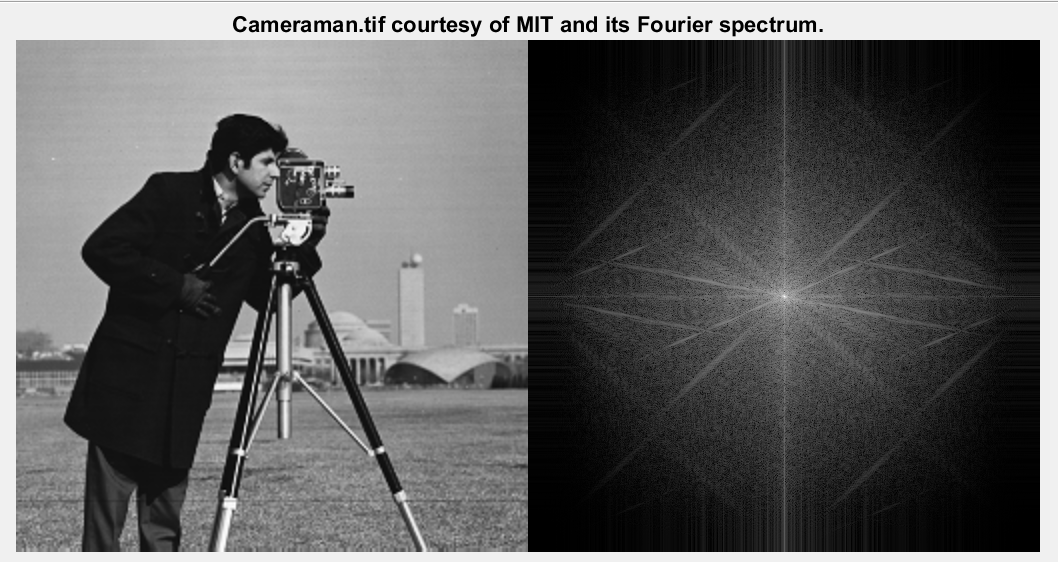Matlab代码：

clear all;
close all;
imshowpair(I,log(abs(fftshift(fft2(I)))+1),'montage')

但是你如果去看matlab的介绍或者在计算FFT之后调用FFTSHIFT函数，那么经过该函数处理过的图像会变成如下形式。如下图。一定要注意：fftshift这一函数的调用是放在傅里叶变换之后的，这和频谱居中的真实计算原理（即，利用傅里叶变换的属性）根本就不一样，可以说是一种投机取巧的方法，但也不失为一个好的方法。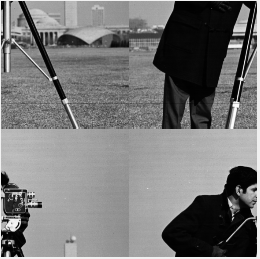Matlab代码：

Ishift = fftshift(I);
imshow(Ishift,[])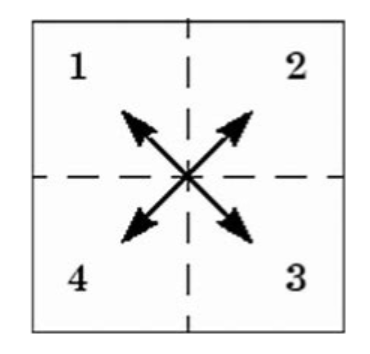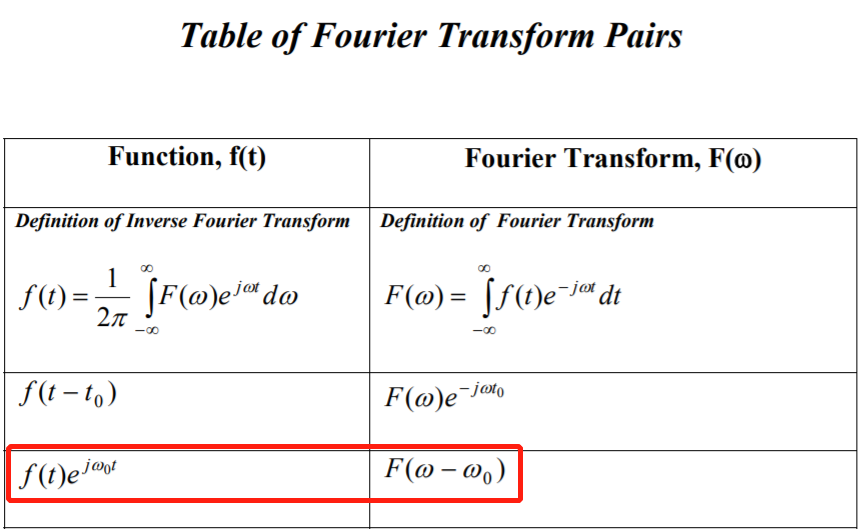要想对频域的信号进行移位，时域的信号需要乘以一个对应的指数函数。这条属性叫做频域移位特性。图像信号在进行变换之前需要对每一个像素乘以（-1）^x+y次方。这是根据欧拉公式计算出来的（注意：e^jπ = -1）。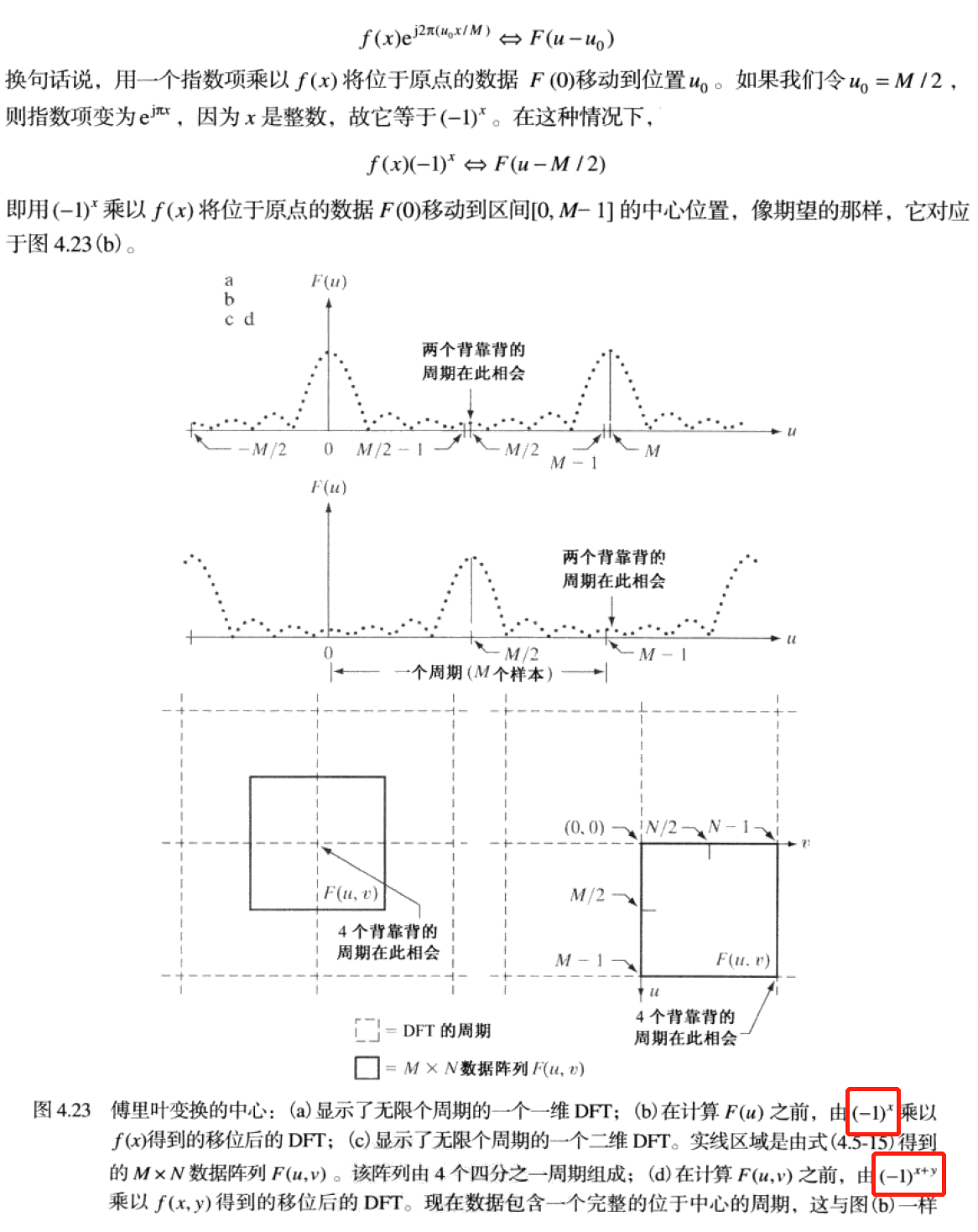Matlab代码：

Ie = zeros(size(I));
for i = 1:size(I,1)
for j = 1:size(I,2)
Ie(i,j) = (-1)^(i+j).*I(i,j);
end
end
imshowpair(I,Ie,'montage')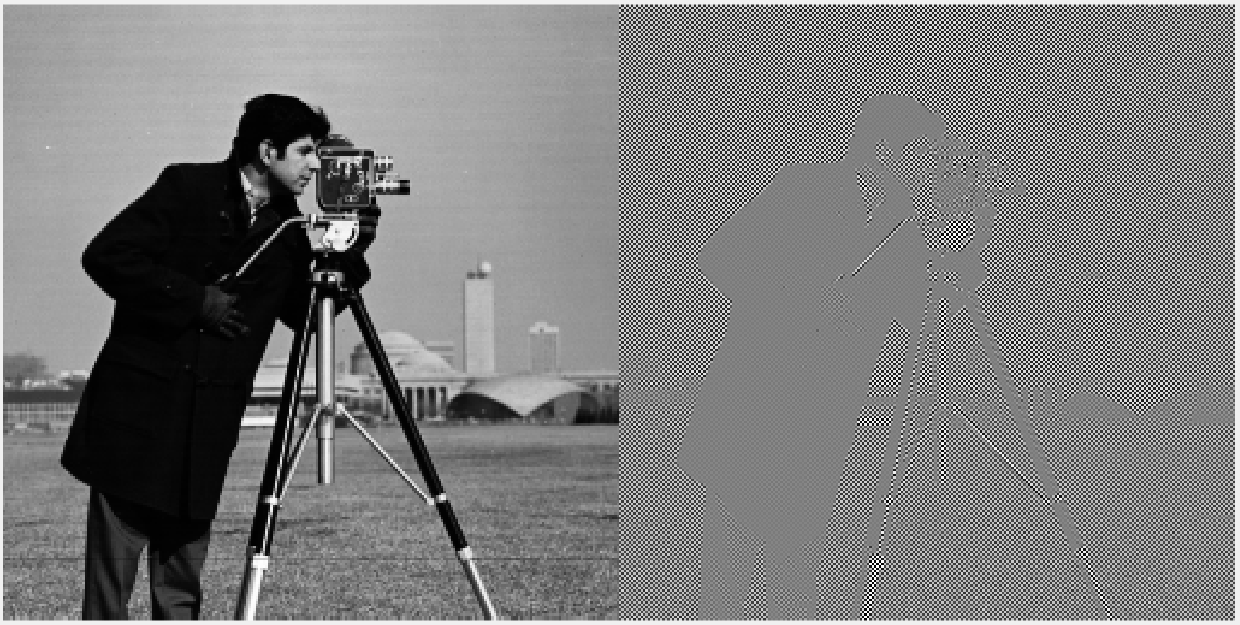简单点说就是一正一负，每隔一个像素乘以一个负号，得到的图像就好像黑白间隔的棋盘一样。如下图放大后的图像细节所示。而不是那种四个象限互换后的结果。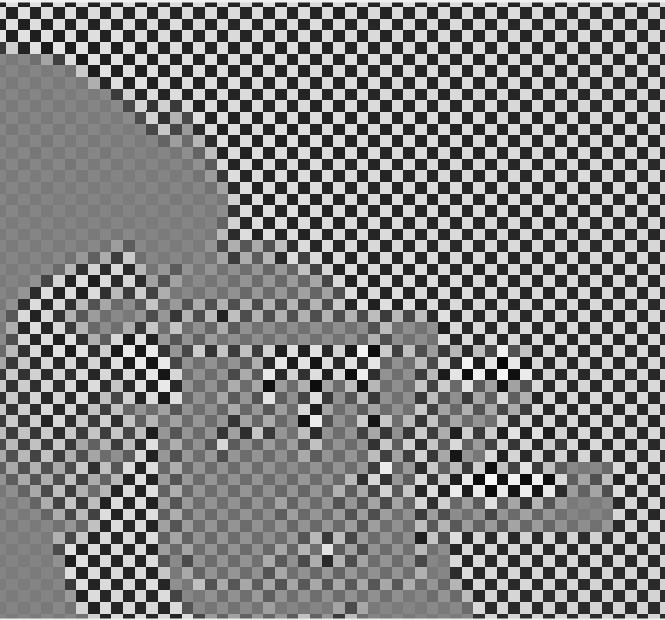最后我用空间域图像乘以指数函数即（-1）^(x+y）的方式对图像的频谱进行居中化的操作，能够得到和MATLAB自带的函数fftshift一模一样的结果。如下图。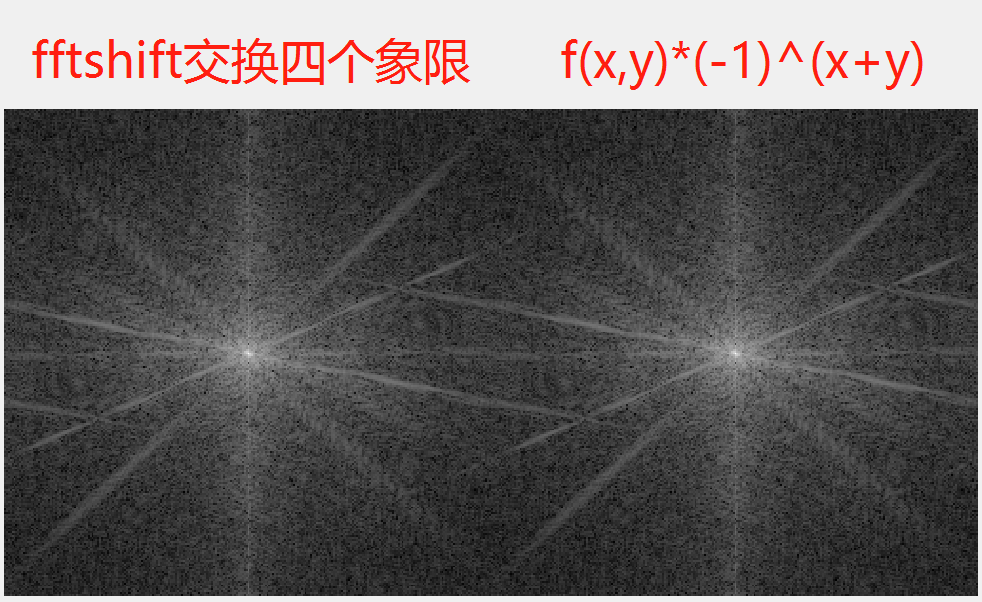Wherefore，不论是一维信号的频谱还是二维信号的频谱，一定要记得真正的频谱居中不只是简单的fftshift或是四个象限的对调而是在进行变换之前，用一个指数函数乘以原始信号。而这个指数函数根据著名的欧拉公式e^jπ+1=0,最终变成了以（-1）为底的幂函数。

（全文完）

【1】Digital image processing, Third Edition.  冈萨雷斯

【2】signals and systems，Second Edition. 奥本海姆

【3】Matlab 2017a

《圣经》箴言 14章12节   有一条路，人以为正，至终成为死亡之路。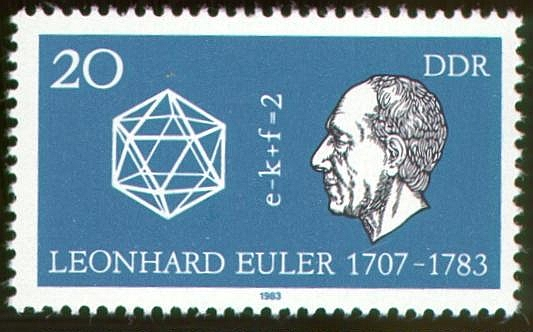*配图与本文无关*

2015-05-21 10:30:12 thnh169 阅读数 10559
• ###### 携手STM32CubeMX玩转STM32

795 人正在学习 去看看 李凯龙

# 1.图像的傅里叶频谱的意义

[数字图像处理]频域滤波(1)–基础与低通滤波器
[数字图像处理]频域滤波(2)–高通滤波器，带阻滤波器与陷波滤波器

# 2. 傅里叶频谱的计算

void cvDFT( const CvArr* src, CvArr* dst, int flags, int nonzero_rows=0 )

|F(u,v)|=R2(u,v)+F2(u,v)2$|F(u,v)|=\sqrt{R^2(u,v) + F^2(u,v)}$

IplImage* fft2(IplImage* image_input)
{
int dftWidth  = getOptimalDFTSize(image_input->width);
int dftHeight = getOptimalDFTSize(image_input->height);

//cout<< " Width" <<  image_input->width << "    " <<  dftWidth  << "\n";
//cout<< "Height" << image_input->height << "    " <<  dftHeight << "\n";

IPL_DEPTH_8U,
1);

IplImage *image_Re =0 , *image_Im = 0, *image_Fourier = 0;

image_Re = cvCreateImage(cvSize(dftWidth,dftHeight),IPL_DEPTH_64F,1);
image_Im = cvCreateImage(cvSize(dftWidth,dftHeight),IPL_DEPTH_64F,1);
image_Fourier = cvCreateImage(cvSize(dftWidth,dftHeight),IPL_DEPTH_64F,2);

//image_Im <--- 0
cvZero(image_Im);
//image_Fourier <--- image_Re
//image_Fourier <--- image_Im
cvMerge(image_Re,image_Im,0,0,image_Fourier);

cvDFT(image_Fourier,image_Fourier,CV_DXT_FORWARD);

//image_Fourier ---> image_Re
//image_Fourier ---> image_Im
cvSplit(image_Fourier,image_Re,image_Im,0,0);

//Mag = sqrt(Re^2 + Im^2)
cvPow(image_Re,image_Re,2.0);
cvPow(image_Im,image_Im,2.0);
cvPow(image_Re,image_Re,0.5);

// log (1 + Mag)
cvLog (image_Re,image_Re);

//  |-----|-----|           |-----|-----|
//  |  1  |  3  |           |  4  |  2  |
//  |-----|-----|   --->    |-----|-----|
//  |  2  |  4  |           |  3  |  1  |
//  |-----|-----|           |-----|-----|

IplImage *Fourier = cvCreateImage(cvSize(dftWidth,dftHeight),IPL_DEPTH_64F,1);
cvZero(image_Fourier);

int cx = image_Re->width/2;
int cy = image_Re->height/2;

cvSetImageROI(image_Re,cvRect( 0, 0,cx,cy));  // 1
cvSetImageROI( Fourier,cvRect(cx,cy,cx,cy));  // 4

cvSetImageROI(image_Re,cvRect(cx,cy,cx,cy));  // 4
cvSetImageROI( Fourier,cvRect( 0, 0,cx,cy));  // 1

cvSetImageROI(image_Re,cvRect(cx, 0,cx,cy));  // 3
cvSetImageROI( Fourier,cvRect( 0,cy,cx,cy));  // 2

cvSetImageROI(image_Re,cvRect( 0,cy,cx,cy));  // 2
cvSetImageROI( Fourier,cvRect(cx, 0,cx,cy));  // 3

cvResetImageROI(image_Re);
cvResetImageROI( Fourier);

cvNormalize(Fourier,Fourier,1,0,CV_C,NULL);

return(Fourier);
}

int dftWidth  = getOptimalDFTSize(image_input->width);
int dftHeight = getOptimalDFTSize(image_input->height);
IPL_DEPTH_8U,
1);
cvCopyMakeBorder( image_input, image_padded, cvPoint(0,0), IPL_BORDER_CONSTANT,cvScalarAll(0)); 

IplImage *image_Re =0 , *image_Im = 0, *image_Fourier = 0;

image_Re = cvCreateImage(cvSize(dftWidth,dftHeight),IPL_DEPTH_64F,1);
image_Im = cvCreateImage(cvSize(dftWidth,dftHeight),IPL_DEPTH_64F,1);
image_Fourier = cvCreateImage(cvSize(dftWidth,dftHeight),IPL_DEPTH_64F,2);

//image_Im <--- 0
cvZero(image_Im);
//image_Fourier <--- image_Re
//image_Fourier <--- image_Im
cvMerge(image_Re,image_Im,0,0,image_Fourier);

cvDFT(image_Fourier,image_Fourier,CV_DXT_FORWARD);

//image_Fourier ---> image_Re
//image_Fourier ---> image_Im
cvSplit(image_Fourier,image_Re,image_Im,0,0);

//Mag = sqrt(Re^2 + Im^2)
cvPow(image_Re,image_Re,2.0);
cvPow(image_Im,image_Im,2.0);
cvPow(image_Re,image_Re,0.5);

// log (1 + Mag)
cvLog (image_Re,image_Re); 

|F(u,v)|=R2(u,v)+F2(u,v)2$|F(u,v)|=\sqrt{R^2(u,v) + F^2(u,v)}$

//  |-----|-----|           |-----|-----|
//  |  1  |  3  |           |  4  |  2  |
//  |-----|-----|   --->    |-----|-----|
//  |  2  |  4  |           |  3  |  1  |
//  |-----|-----|           |-----|-----|

IplImage *Fourier = cvCreateImage(cvSize(dftWidth,dftHeight),IPL_DEPTH_64F,1);
cvZero(image_Fourier);

int cx = image_Re->width/2;
int cy = image_Re->height/2;

cvSetImageROI(image_Re,cvRect( 0, 0,cx,cy));  // 1
cvSetImageROI( Fourier,cvRect(cx,cy,cx,cy));  // 4

cvSetImageROI(image_Re,cvRect(cx,cy,cx,cy));  // 4
cvSetImageROI( Fourier,cvRect( 0, 0,cx,cy));  // 1

cvSetImageROI(image_Re,cvRect(cx, 0,cx,cy));  // 3
cvSetImageROI( Fourier,cvRect( 0,cy,cx,cy));  // 2

cvSetImageROI(image_Re,cvRect( 0,cy,cx,cy));  // 2
cvSetImageROI( Fourier,cvRect(cx, 0,cx,cy));  // 3

cvResetImageROI(image_Re);
cvResetImageROI( Fourier);

cvNormalize(Fourier,Fourier,1,0,CV_C,NULL);

return(Fourier);

[数字图像处理]频域滤波(1)–基础与低通滤波器# 3. 不用交换操作的代码

f(x,y)=f(x,y)×(1)x+y$f(x,y)=f(x,y)\times(-1)^{x+y}$

[数字图像处理]频域滤波(1)–基础与低通滤波器

IplImage* fft2_New(IplImage* image_input)
{
int dftWidth  = getOptimalDFTSize(image_input->width);
int dftHeight = getOptimalDFTSize(image_input->height);

cout<< " Width" <<  image_input->width << "    " <<  dftWidth  << "\n";
cout<< "Height" << image_input->height << "    " <<  dftHeight << "\n";

IPL_DEPTH_8U,
1);

IplImage *image_Re =0 , *image_Im = 0, *image_Fourier = 0;

image_Re = cvCreateImage(cvSize(dftWidth,dftHeight),IPL_DEPTH_64F,1);
image_Im = cvCreateImage(cvSize(dftWidth,dftHeight),IPL_DEPTH_64F,1);
image_Fourier = cvCreateImage(cvSize(dftWidth,dftHeight),IPL_DEPTH_64F,2);

double pixel;
{
{
pixel = ((x+y)%2 == 0)?(pixel):((-1)*pixel);
cvSetReal2D(image_Re,x,y,pixel);
}
}

//image_Im <--- 0
cvZero(image_Im);
//image_Fourier <--- image_Re
//image_Fourier <--- image_Im
cvMerge(image_Re,image_Im,0,0,image_Fourier);

cvDFT(image_Fourier,image_Fourier,CV_DXT_FORWARD);

//image_Fourier ---> image_Re
//image_Fourier ---> image_Im
cvSplit(image_Fourier,image_Re,image_Im,0,0);

//Mag = sqrt(Re^2 + Im^2)
cvPow(image_Re,image_Re,2.0);
cvPow(image_Im,image_Im,2.0);
cvPow(image_Re,image_Re,0.5);

// log (1 + Mag)
cvLog (image_Re,image_Re);

cvNormalize(image_Re,image_Re,1,0,CV_C,NULL);

return(image_Re);
}

for(int y=0;y<image_padded->height;y++)
{
{
pixel = ((x+y)%2 == 0)?(pixel):((-1)*pixel);
cvSetReal2D(image_Re,x,y,pixel);
}
}1. 对于原图而言两个函数的结果基本一致，两个函数都得到了正确的结果。
2. 使用平滑处理后，频谱的高频成分明显变小，对于空间域的图像而言，图像变得模糊了。

## 参考文献

opencv 中 傅里叶变换 FFT :http://blog.csdn.net/abcjennifer/article/details/7359952
OpenCV实现基于傅里叶变换的旋转文本校正 : http://johnhany.net/2013/11/dft-based-text-rotation-correction/#imageclose-380
学习OpenCV范例（八）——离散傅立叶变换 : http://blog.csdn.net/chenjiazhou12/article/details/21240647

## =============更新日志===================

2015 - 5 - 19 初版
2015 - 5 - 21 修改了某些叙述

2019-07-06 10:42:52 qq_33208851 阅读数 1847
• ###### 携手STM32CubeMX玩转STM32

795 人正在学习 去看看 李凯龙

• 频域(frequency domain)
是指在对函数或信号进行分析时，分析其和频率有关部份，而不是和时间有关的部份，和时域一词相对。
• 时域
是描述数学函数或物理信号对时间的关系。例如一个信号的时域波形可以表达信号随着时间的变化。若考虑离散时间，时域中的函数或信号，在各个离散时间点的数值均为已知。若考虑连续时间，则函数或信号在任意时间的数值均为已知。在研究时域的信号时，常会用示波器将信号转换为其时域的波形。
• 两者相互间的变换
时域（信号对时间的函数）和频域（信号对频率的函数）的变换在数学上是通过积分变换实现。对周期信号可以直接使用傅立叶变换，对非周期信号则要进行周期扩展，使用拉普拉斯变换。

• 图像高频分量：图像突变部分；在某些情况下指图像边缘信息，某些情况 下指噪声，更多是两者的混合；
• 低频分量：图像变化平缓的部分，也就是图像轮廓信息
• 高通滤波器：让图像使低频分量抑制，高频分量通过
• 低通滤波器：与高通相反，让图像使高频分量抑制，低频分量通过
• 带通滤波器：使图像在某一部分 的频率信息通过，其他过低或过高都抑制
• 还有个带阻滤波器，是带通的反。

## 1. 傅里叶变换及其反变换

### 1.0 什么是傅里叶变换

1. 什么是傅里叶变换？
也称作傅立叶变换，表示能将满足一定条件的某个函数表示成三角函数（正弦和/或余弦函数）或者它们的积分的线性组合。在不同的研究领域，傅立叶变换具有多种不同的变体形式，如连续傅立叶变换和离散傅立叶变换。傅里叶变换是一种分析信号的方法，它可分析信号的成分，也可用这些成分合成信号。许多波形可作为信号的成分，比如正弦波、方波、锯齿波等，傅里叶变换用正弦波作为信号的成分。
傅里叶变换的实质是将一个信号分离为无穷多多正弦/复指数信号的加成，也就是说，把信号变成正弦信号相加的形式——既然是无穷多个信号相加，那对于非周期信号来说，每个信号的加权应该都是零——但有密度上的差别，你可以对比概率论中的概率密度来思考一下——落到每一个点的概率都是无限小，但这些无限小是有差别的所以，傅里叶变换之后，横坐标即为分离出的正弦信号的频率，纵坐标对应的是加权密度
2. 傅里叶变换有什么用呢？
举例说明：傅里叶变换可以将一个时域信号转换成在不同频率下对应的振幅及相位，其频谱就是时域信号在频域下的表现，而反傅里叶变换可以将频谱再转换回时域的信号。最简单最直接的应用就是时频域转换，比如在移动通信的LTE系统中，要把接收的信号从时域变成频域，就需要使用FFT（快速傅里叶变换）。又例如对一个采集到的声音做傅立叶变化就能分出好几个频率的信号。比如南非世界杯时，南非人吹的呜呜主拉的声音太吵了，那么对现场的音频做傅立叶变化（当然是对声音的数据做），会得到一个展开式，然后找出呜呜主拉的特征频率，去掉展开式中的那个频率的sin函数，再还原数据，就得到了没有呜呜主拉的嗡嗡声的现场声音。而对图片的数据做傅立叶，然后增大高频信号的系数就可以提高图像的对比度。同样，相机自动对焦就是通过找图像的高频分量最大的时候，就是对好了。

### 1.1 为什么要在频率域研究图像增强？

• 可以利用频率成分和图像外表之间的对应关系。一些在空间域表述困难的增强任务，在频率域中变得非常普通
• 滤波在频率域更为直观，它可以解释空间域滤波的某些性质
• 可以在频率域指定滤波器，做反变换，然后在空间域使用结果滤波器作为空间域滤波器的指导
• 一旦通过频率域试验选择了空间滤波，通常实施都在空间域进行

### 1.2 傅里叶变换及反转

#### 1.2.1 一维连续傅里叶变换及反变换#### 1.2.2 二维连续傅里叶变换及反变换F(u,v) = F(− u,−v)

|F(u,v)| =| F(− u,−v)|#### 1.2.3 一维离散傅里叶变换及反变换#### 1.2.4 二维离散傅里叶变换及反变换u=0,1,2,…,M-1, v=0,1,2,…,N-1x=0,1,2,…,M-1, y=0,1,2,…,N-1

F(0,0)表示：#### 1.2.5 傅里叶变换的一维极坐标表示R(u)和I(u)分别是F(u)的实部和虚部f(x)的离散表示：F(u)的离散表示：#### 1.2.6 傅里叶变换的二维极坐标表示R(u,v)和I(u,v)分别是F(u,v)的实部和虚部F(u,v)的原点变换：u=0,1,2,…,M-1, v=0,1,2,…,N-1

## 2. 傅里叶变换的性质

### 2.1 平移性### 2.2 分配律### 2.3 尺度变换（缩放）### 2.4 旋转性f(x,y)旋转角度θ 0，F(u,v)也将转过相同的角度
F(u,v)旋转角度θ 0，f(x,y)也将转过相同的角度

### 2.5 周期性和共轭对称性### 2.6 分离性F(x,v)是沿着f(x,y)的一行所进行的傅里叶变换。当x=0,1,…,M-1，沿着f(x,y)的所有行计算傅里叶变换。 先通过沿输入图像的每一行计算一维变换
 再沿中间结果的每一列计算一维变换
 可以改变上述顺序，即先列后行
 上述相似的过程也可以计算二维傅里叶反变换

### 2.7 平均值### 2.8 卷积理论### 2.9 相关性理论

 f(x,y)是原始图像
 h(x,y)作为感兴趣的物体或区域（模板）
 如果匹配，两个函数的相关值会在h找到f中相应点的位置上达到最大f* 表示f的复共轭。对于实函数，f*＝f## 3. 快速傅里叶变换（FFT）

### 3.1 为什么需要快速傅里叶变换？• 对u的M个值中的每一个都需进行M次复数乘法(将f(x)与 e− j2πux / M 相乘)和M-1次加法，即复数乘法和加法的次数都正比于M2
• 快速傅里叶变换(FFT)则只需要Mlog2M次运算
• FFT算法与原始变换算法的计算量之比是log2M/M，如M=1024≈103,则原始变换算法需要106次计算，而FFT需 要104次计算，FFT与原始变换算法之比是1：100
只考虑一维的情况，根据傅里叶变换的分离性可知，二维傅里叶变换可由连续2次一维傅里叶变换得到

### 3.2 FFT算法基本思想

FFT算法基于一个叫做逐次加倍的方法。通过推导将原始傅里叶转换成两个递推公式：### 3.3 FFT公式推导M = 2n， n为正整数。因此，M可以表示为：M = 2K 。将M=2K带入上式：• 一个M个点的变换，能够通过将原始表达式分成两个部分来计算
• 通过计算两个（M/2）个点的变换。得Feven(u)和 Fodd(u)
• 奇部与偶部之和得到F(u)的前(M/2)个值
• 奇部与偶部之差得到F(u)的后(M/2)个值。且不需要额外的变换计算

### 3.4 归纳快速傅立叶变换的思想

（1）通过计算两个单点的DFT，来计算两个点的DFT， （2）通过计算两个双点的DFT，来计算四个点的DFT，…，以此类推
（3）对于任何N=2m的DFT的计算，通过计算两个N/2点的DFT，来计算N个点的DFT

### 3.5 FFT算法举例

{F(0),F(1),F(2),F(3),F(4),F(5),F(6),F(7)}

{ f(0), f(2), f(4), f(6) }
{ f(1), f(3), f(5), f(7) }

{ f(0), f(4) }， { f(2), f(6) }
{ f(1), f(5) }， { f(3), f(7) }2）计算顺序及地址增量：2n, n = 0,1,2…## 4. 傅里叶变换的物理意义

1. 图像的频率是表征图像中灰度变化剧烈程度的指标，是灰度在平面空间上的梯度（灰度变化得快频率就高，灰度变化得慢频率就低）。如：大面积的沙漠在图像中是一片灰度变化缓慢的区域，对应的频率值很低；而对于地表属性变换剧烈的边缘区域在图像中是一片灰度变化剧烈的区域，对应的频率值较高。傅立叶变换在实际中有非常明显的物理意义，设f是一个能量有限的模拟信号，则其傅立叶变换就表示f的谱。从纯粹的数学意义上看，傅立叶变换是将一个函数转换为一系列周期函数来处理的。从物理效果看，傅立叶变换是将图像从空间域转换到频率域，其逆变换是将图像从频率域转换到空间域。换句话说，傅立叶变换的物理意义是将图像的灰度分布函数变换为图像的频率分布函数，傅立叶逆变换是将图像的频率分布函数变换为灰度分布函数
2. 傅立叶变换以前，图像（未压缩的位图）是由对在连续空间（现实空间）上的采样得到一系列点的集合，我们习惯用一个二维矩阵表示空间上各点，则图像可由z=f(x,y)来表示。由于空间是三维的，图像是二维的，因此空间中物体在另一个维度上的关系就由梯度来表示，这样我们可以通过观察图像得知物体在三维空间中的对应关系。为什么要提梯度？因为实际上对图像进行二维傅立叶变换得到频谱图，就是图像梯度的分布图，当然频谱图上的各点与图像上各点并不存在一一对应的关系，即使在不移频的情况下也是没有。傅立叶频谱图上我们看到的明暗不一的亮点，实际上图像上某一点与邻域点灰度值差异的强弱，即梯度的大小，也即该点的频率的大小（差异/梯度越大，频率越高，能量越低，在频谱图上就越 暗。差异/梯度越小，频率越低，能量越高，在频谱图上就越 亮。换句话说，频率谱上越亮能量越高，频率越低，图像差异越小/平缓）。一般来讲，梯度大则该点的亮度强，否则该点亮度弱频谱图，也叫功率图在经过频谱中心化（用(-1)x+y乘以输入的图像函数）后的频谱中，中间最亮的点是最低频率，属于直流分量（DC分量）(当频率为0时，表示直流信号，没有变化。在原点(u,v两个频率域变量均为零)的傅里叶变换即等于图像的平均灰度级,F(0，0)称做频 率谱的直流成分)。越往边外走，频率越高。所以，频谱图中的四个角和X,Y轴的尽头都是高频，如下图：